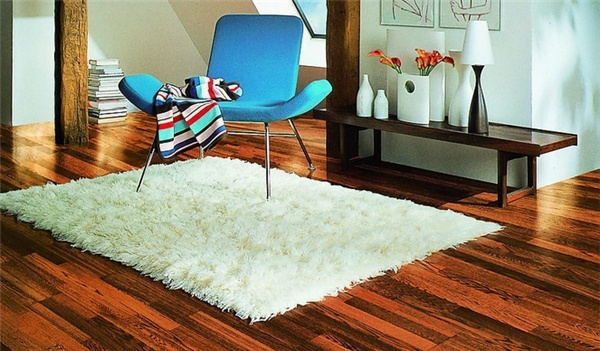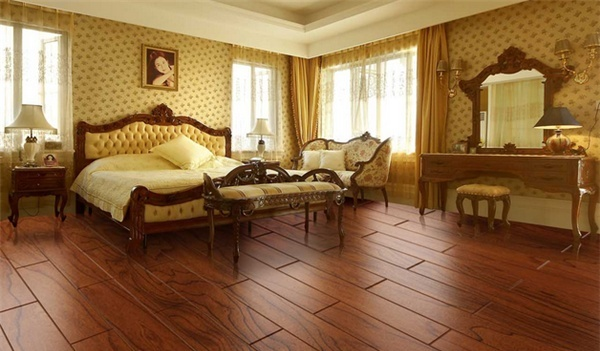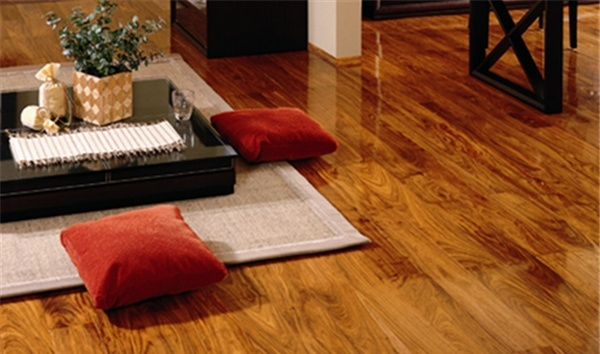|

# 地暖用什么地板 适合地暖的地板品牌

地暖地板是当前所有的地板生产企业研究的重大课题，处于环保的要求，地暖已经成为当前众多家庭采暖的首要选择方案，因此对于地暖地板的要求自然也就提上了日程。对地暖用什么地板也成为安装了地暖家庭装修时的研究课题，许多业主根本就不知道适合地暖的地板有哪些，下面就给大家介绍一些地暖用什么地板好。地暖用什么地板

一、甲醛释放量不能超标

要求在长时间加热的条件下，甲醛释放量不会超标。消费者选择地热地板时，一定要尽可能选择甲醛含量低的地板，因为温度越高，甲醛释放量越多，在相对封闭的居室内，再加上室内其他物品造成的甲醛释放，室内甲醛有可能会超标，对人体造成危害。

二、尺寸稳定性要好

这一点要看地板的基材密度及内结合强度，内结合强度越高，说明地板承受温度变化的能力越好，不至于发生开裂等现象，只有基材为相对致密的木材的地板，才能保证长期在高温下不开裂、不变形。不惧潮湿环境即吸水厚度膨胀率。用于水热地面辐射采暖的地板要求在高湿状态下尺寸变化小，膨胀率要小于等于2。5％。一般来说，膨胀率越小，地板的防潮性能越好。

三、传热要快

目前市场上能提供热传导系数的品牌不多，消费者在购买时要留意。一般系数高的产品在导热方面性能更好。不怕高温由于地热地板要长时间承受高温加热，要求产品装饰层性能稳定，在长时间高温的条件下也不会出现褪色、糙光的现象复合地板更适合地热采暖一般来说，进口地板质量达到欧洲Enpr13329标准，国产地板质量达到国家GB／T1802－2000标准，都可以适应地热采暖地板安装的技术要求。可能看了以上这些笼统的标准，有的读者还是不知道应该选择什么样的产品。

现在市场上地板种类很多，有实木地板、复合地板等多种，一般来说，复合地板更适合用做地热地板。因为地热地板的标准厚度为6.5～8.5mm，复合地板的厚度大多为6～9mm，其中强化复合地板多为6～8mm，三层实木复合地板为8～9mm，传热性能更好，且其表面有一层三氧化二铝的耐磨层，这个耐磨层有利于热量在地表快速扩散。适合地暖的地板品牌

1圣象Power Dekor（ 曾获中国名牌，地板十大品牌，极具知名度的家居建材品牌之一，致力于研发生产各类地板的企业，圣象集团有限公司 ）

2安心地板（ 地板十大品牌，行业标志性品牌，中国品牌500强，具有深厚文化底蕴的木地板企业，浙江安心木业有限公司 ）

3安信（ 上海市着名商标，上海市名牌，中国500最具价值品牌，上海木材行业协会会长单位，安信伟光（上海）木材有限公司 ）

4柏尔Boer（ 始于1996年，相关行业标准起草单位，双锁扣地板行业领导者，十大地热地板品牌，浙江柏尔木业有限公司 ）

5生活家（ 全球最大的仿古地板生产企业之一，致力于满足全球消费者居家木制品需求的企业，巴洛克木业（中山）有限公司 ）

6大自然Nature（ 中国500最具价值品牌，曾获中国名牌，地板行业国家标准起草单位，十大木地板品牌，大自然家居（中国）有限公司 ）

7柏金地板（ 燕加隆旗下，高端实木地热锁扣地板领导者，中国实木地热地板生产示范企业，深圳市森诺韦尔建材有限公司 ）

8瑞嘉WELLUX（ 品牌500强，地采暖用木质地板标准起草单位，中国环境标志产品，中国林产工业协会地板委员会副理事长单位，北京瑞嘉欧亚木业有限公司 ）

9久盛TREESSUN（ 高新技术企业，浙江名牌产品，实木地板十大品牌，国内最专业的大型木地板制造企业之一，浙江久盛地板有限公司 ）

10宏耐HOMENICE（ 曾获中国名牌，江苏省着名商标，中国品牌500强，中国最具影响力的建材品牌之一，南京宏耐木业有限公司 ）地暖安装误区有哪些？

误区1：水地暖湿润、电地暖干燥

很多人以为水地暖湿润而电地暖干燥，其实不是这样的。水地暖的水是加热的载体，并不会用来调节湿度的。而发热电缆，是直接将电能转化为热能。这些热被混凝土层和地板层吸收后加热房间。

误区2：铜制散热器不腐蚀

业主一般认为，铜和铝比钢的耐腐蚀性能都要好，所以不会发生腐蚀，但在一定环境下，铜制散热器也会因系统中如果有淤泥、含硫量过多，或者选用的铜表面附有碳化层等造成铜制散热器的腐蚀。铝制散热器容易因供热系统的水质呈碱性、或氯离子含量较高而腐蚀。

误区3：内涂等于防腐

在散热器市场，销售人员言必称“防腐”。其实截至目前为止国内散热器市场上常见的内涂防腐类型不外乎两种：“有机涂层”和“无机涂层”。一般来讲，无机涂料成本高于有机涂料，流程更复杂，工艺要求更严格，防腐效果也好于有机涂料。

误区4：名牌产品在哪儿买都一样

据资料显示，在小区里临时销售点购买散热器是最容易出问题的。因为很多消费者使用一段时间后出了问题反映到厂家，厂家却发现散热器品牌是假冒的，回头再找经销商也不见了，消费者最终只能认倒霉。友情提示：地暖地板是地暖采暖中重要的一部分，地板不仅关系家庭采暖还与装修建材相关，在地暖项目中推荐用户采用复合地板，这样既可以保证地暖的使用效果，也可以避免地暖在使用过程中带来的一些问题，让地暖更好的为我们服务。

`声明：本文由入驻焦点开放平台的作者撰写，除焦点官方账号外，观点仅代表作者本人，不代表焦点立场错误信息举报电话： 400-099-0099，邮箱：jubao@vip.sohu.com，或点此进行意见反馈，或点此进行举报投诉。`A B C D E F G H J K L M N P Q R S T W X Y Z
A - B - C - D - E
• A
• 鞍山
• 安庆
• 安阳
• 安顺
• 安康
• 澳门
• B
• 北京
• 保定
• 包头
• 巴彦淖尔
• 本溪
• 蚌埠
• 亳州
• 滨州
• 北海
• 百色
• 巴中
• 毕节
• 保山
• 宝鸡
• 白银
• 巴州
• C
• 承德
• 沧州
• 长治
• 赤峰
• 朝阳
• 长春
• 常州
• 滁州
• 池州
• 长沙
• 常德
• 郴州
• 潮州
• 崇左
• 重庆
• 成都
• 楚雄
• 昌都
• 慈溪
• 常熟
• D
• 大同
• 大连
• 丹东
• 大庆
• 东营
• 德州
• 东莞
• 德阳
• 达州
• 大理
• 德宏
• 定西
• 儋州
• 东平
• E
• 鄂尔多斯
• 鄂州
• 恩施
F - G - H - I - J
• F
• 抚顺
• 阜新
• 阜阳
• 福州
• 抚州
• 佛山
• 防城港
• G
• 赣州
• 广州
• 桂林
• 贵港
• 广元
• 广安
• 贵阳
• 固原
• H
• 邯郸
• 衡水
• 呼和浩特
• 呼伦贝尔
• 葫芦岛
• 哈尔滨
• 黑河
• 淮安
• 杭州
• 湖州
• 合肥
• 淮南
• 淮北
• 黄山
• 菏泽
• 鹤壁
• 黄石
• 黄冈
• 衡阳
• 怀化
• 惠州
• 河源
• 贺州
• 河池
• 海口
• 红河
• 汉中
• 海东
• 怀来
• I
• J
• 晋中
• 锦州
• 吉林
• 鸡西
• 佳木斯
• 嘉兴
• 金华
• 景德镇
• 九江
• 吉安
• 济南
• 济宁
• 焦作
• 荆门
• 荆州
• 江门
• 揭阳
• 金昌
• 酒泉
• 嘉峪关
K - L - M - N - P
• K
• 开封
• 昆明
• 昆山
• L
• 廊坊
• 临汾
• 辽阳
• 连云港
• 丽水
• 六安
• 龙岩
• 莱芜
• 临沂
• 聊城
• 洛阳
• 漯河
• 娄底
• 柳州
• 来宾
• 泸州
• 乐山
• 六盘水
• 丽江
• 临沧
• 拉萨
• 林芝
• 兰州
• 陇南
• M
• 牡丹江
• 马鞍山
• 茂名
• 梅州
• 绵阳
• 眉山
• N
• 南京
• 南通
• 宁波
• 南平
• 宁德
• 南昌
• 南阳
• 南宁
• 内江
• 南充
• P
• 盘锦
• 莆田
• 平顶山
• 濮阳
• 攀枝花
• 普洱
• 平凉
Q - R - S - T - W
• Q
• 秦皇岛
• 齐齐哈尔
• 衢州
• 泉州
• 青岛
• 清远
• 钦州
• 黔南
• 曲靖
• 庆阳
• R
• 日照
• 日喀则
• S
• 石家庄
• 沈阳
• 双鸭山
• 绥化
• 上海
• 苏州
• 宿迁
• 绍兴
• 宿州
• 三明
• 上饶
• 三门峡
• 商丘
• 十堰
• 随州
• 邵阳
• 韶关
• 深圳
• 汕头
• 汕尾
• 三亚
• 三沙
• 遂宁
• 山南
• 商洛
• 石嘴山
• T
• 天津
• 唐山
• 太原
• 通辽
• 铁岭
• 泰州
• 台州
• 铜陵
• 泰安
• 铜仁
• 铜川
• 天水
• 天门
• W
• 乌海
• 乌兰察布
• 无锡
• 温州
• 芜湖
• 潍坊
• 威海
• 武汉
• 梧州
• 渭南
• 武威
• 吴忠
• 乌鲁木齐
X - Y - Z
• X
• 邢台
• 徐州
• 宣城
• 厦门
• 新乡
• 许昌
• 信阳
• 襄阳
• 孝感
• 咸宁
• 湘潭
• 湘西
• 西双版纳
• 西安
• 咸阳
• 西宁
• 仙桃
• 西昌
• Y
• 运城
• 营口
• 盐城
• 扬州
• 鹰潭
• 宜春
• 烟台
• 宜昌
• 岳阳
• 益阳
• 永州
• 阳江
• 云浮
• 玉林
• 宜宾
• 雅安
• 玉溪
• 延安
• 榆林
• 银川
• Z
• 张家口
• 镇江
• 舟山
• 漳州
• 淄博
• 枣庄
• 郑州
• 周口
• 驻马店
• 株洲
• 张家界
• 珠海
• 湛江
• 肇庆
• 中山
• 自贡
• 资阳
• 遵义
• 昭通
• 张掖
• 中卫

1室1厅1厨1卫1阳台

1
2
3
4
5

0
1
2

1

1

0
1
2
3报名成功，资料已提交审核A B C D E F G H J K L M N P Q R S T W X Y Z
A - B - C - D - E
• A
• 鞍山
• 安庆
• 安阳
• 安顺
• 安康
• 澳门
• B
• 北京
• 保定
• 包头
• 巴彦淖尔
• 本溪
• 蚌埠
• 亳州
• 滨州
• 北海
• 百色
• 巴中
• 毕节
• 保山
• 宝鸡
• 白银
• 巴州
• C
• 承德
• 沧州
• 长治
• 赤峰
• 朝阳
• 长春
• 常州
• 滁州
• 池州
• 长沙
• 常德
• 郴州
• 潮州
• 崇左
• 重庆
• 成都
• 楚雄
• 昌都
• 慈溪
• 常熟
• D
• 大同
• 大连
• 丹东
• 大庆
• 东营
• 德州
• 东莞
• 德阳
• 达州
• 大理
• 德宏
• 定西
• 儋州
• 东平
• E
• 鄂尔多斯
• 鄂州
• 恩施
F - G - H - I - J
• F
• 抚顺
• 阜新
• 阜阳
• 福州
• 抚州
• 佛山
• 防城港
• G
• 赣州
• 广州
• 桂林
• 贵港
• 广元
• 广安
• 贵阳
• 固原
• H
• 邯郸
• 衡水
• 呼和浩特
• 呼伦贝尔
• 葫芦岛
• 哈尔滨
• 黑河
• 淮安
• 杭州
• 湖州
• 合肥
• 淮南
• 淮北
• 黄山
• 菏泽
• 鹤壁
• 黄石
• 黄冈
• 衡阳
• 怀化
• 惠州
• 河源
• 贺州
• 河池
• 海口
• 红河
• 汉中
• 海东
• 怀来
• I
• J
• 晋中
• 锦州
• 吉林
• 鸡西
• 佳木斯
• 嘉兴
• 金华
• 景德镇
• 九江
• 吉安
• 济南
• 济宁
• 焦作
• 荆门
• 荆州
• 江门
• 揭阳
• 金昌
• 酒泉
• 嘉峪关
K - L - M - N - P
• K
• 开封
• 昆明
• 昆山
• L
• 廊坊
• 临汾
• 辽阳
• 连云港
• 丽水
• 六安
• 龙岩
• 莱芜
• 临沂
• 聊城
• 洛阳
• 漯河
• 娄底
• 柳州
• 来宾
• 泸州
• 乐山
• 六盘水
• 丽江
• 临沧
• 拉萨
• 林芝
• 兰州
• 陇南
• M
• 牡丹江
• 马鞍山
• 茂名
• 梅州
• 绵阳
• 眉山
• N
• 南京
• 南通
• 宁波
• 南平
• 宁德
• 南昌
• 南阳
• 南宁
• 内江
• 南充
• P
• 盘锦
• 莆田
• 平顶山
• 濮阳
• 攀枝花
• 普洱
• 平凉
Q - R - S - T - W
• Q
• 秦皇岛
• 齐齐哈尔
• 衢州
• 泉州
• 青岛
• 清远
• 钦州
• 黔南
• 曲靖
• 庆阳
• R
• 日照
• 日喀则
• S
• 石家庄
• 沈阳
• 双鸭山
• 绥化
• 上海
• 苏州
• 宿迁
• 绍兴
• 宿州
• 三明
• 上饶
• 三门峡
• 商丘
• 十堰
• 随州
• 邵阳
• 韶关
• 深圳
• 汕头
• 汕尾
• 三亚
• 三沙
• 遂宁
• 山南
• 商洛
• 石嘴山
• T
• 天津
• 唐山
• 太原
• 通辽
• 铁岭
• 泰州
• 台州
• 铜陵
• 泰安
• 铜仁
• 铜川
• 天水
• 天门
• W
• 乌海
• 乌兰察布
• 无锡
• 温州
• 芜湖
• 潍坊
• 威海
• 武汉
• 梧州
• 渭南
• 武威
• 吴忠
• 乌鲁木齐
X - Y - Z
• X
• 邢台
• 徐州
• 宣城
• 厦门
• 新乡
• 许昌
• 信阳
• 襄阳
• 孝感
• 咸宁
• 湘潭
• 湘西
• 西双版纳
• 西安
• 咸阳
• 西宁
• 仙桃
• 西昌
• Y
• 运城
• 营口
• 盐城
• 扬州
• 鹰潭
• 宜春
• 烟台
• 宜昌
• 岳阳
• 益阳
• 永州
• 阳江
• 云浮
• 玉林
• 宜宾
• 雅安
• 玉溪
• 延安
• 榆林
• 银川
• Z
• 张家口
• 镇江
• 舟山
• 漳州
• 淄博
• 枣庄
• 郑州
• 周口
• 驻马店
• 株洲
• 张家界
• 珠海
• 湛江
• 肇庆
• 中山
• 自贡
• 资阳
• 遵义
• 昭通
• 张掖
• 中卫• 手机• 分享
• 设计
免费设计
• 计算器
装修计算器
• 入驻
合作入驻
• 联系
联系我们
• 置顶
返回顶部